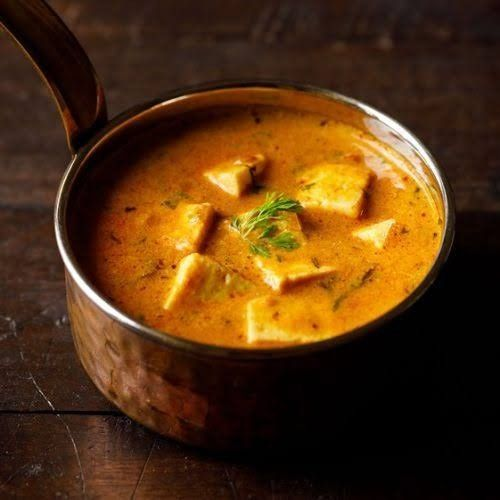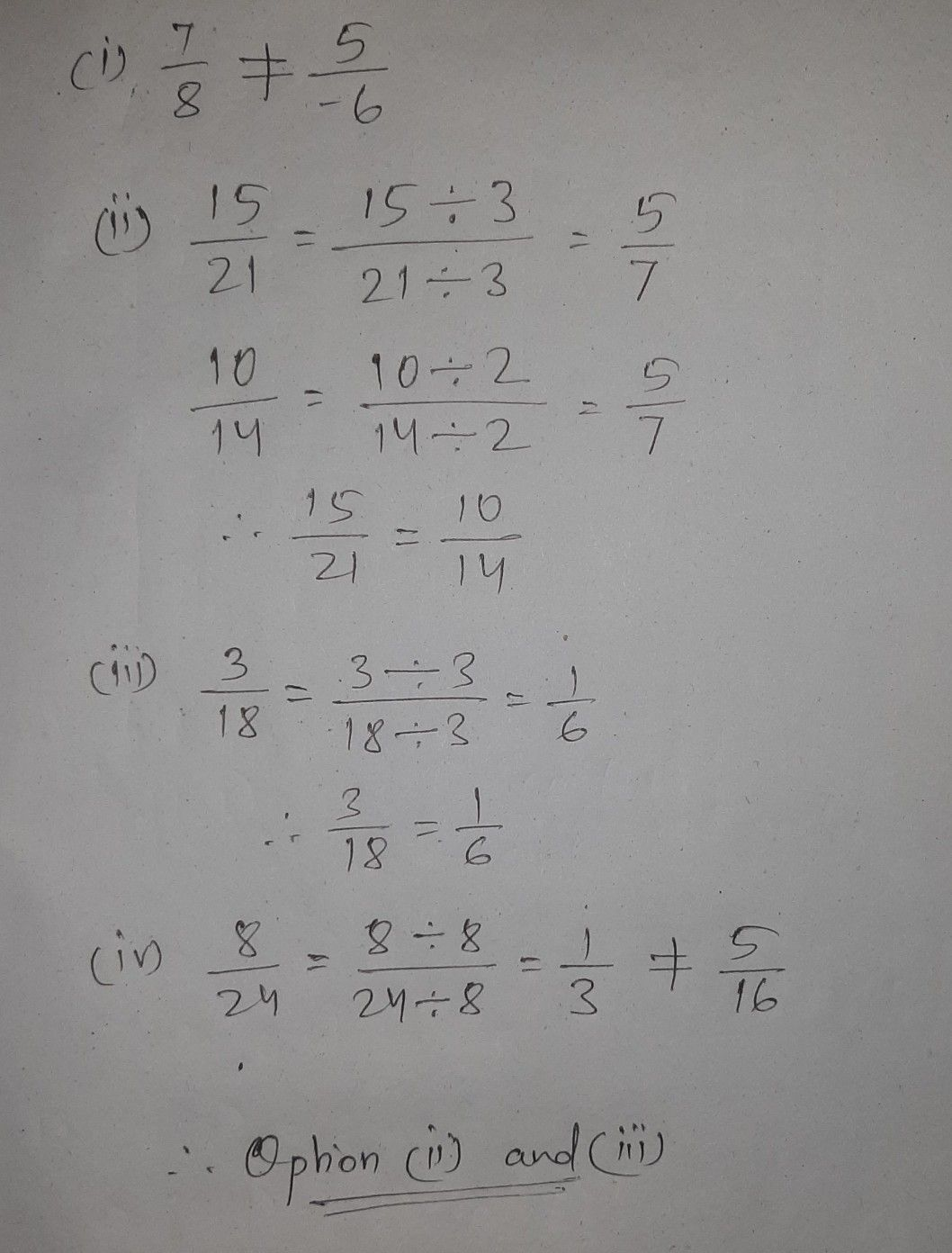Symbol
Problem$8$ Which of the following pairs of rational numbers are equivalent? $\left(i\right)$ $\dfrac {7} {8}$ $\dfrac {5} {-6}$ $\left(ii\right)$ $\dfrac {15} {21},\dfrac {10} {14}$ $\left(iii\right)$ $\dfrac {3} {18}$ $\dfrac {1} {6}$ $\left(iv\right)$ $\dfrac {8} {24}$ $\dfrac {5} {16}$ $Ex$ $mross$ following rational numbers in the sta ard form.
7th-9th grade
Other
SolutionQanda teacher - BhanuStudent
it is not the correct answer
first and second are equivalentQanda teacher - Bhanu
please check the solution
in the first bit
one fraction is negative
and other is positive
how they can be equivalentStudent
but in my book I first and second are equivalent